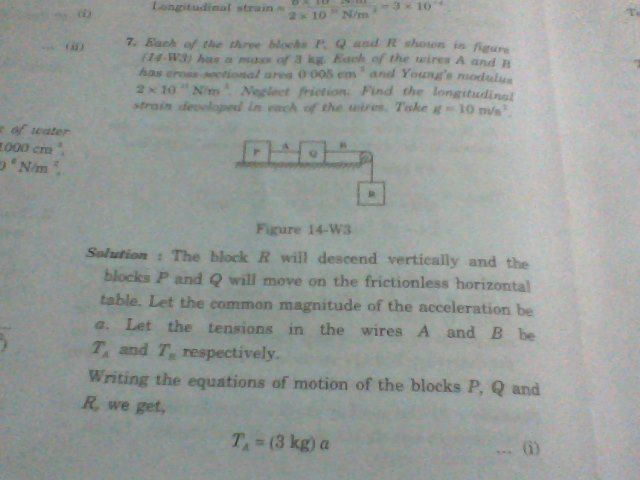# Why & how do bodies attached by extensible strings move with common acceleration?

From the very beginning of my journey of physics, I have been taught that while dealing problems having attachment of one body to another mass ,

if the string connecting the bodies be inextensible , then only all the bodies connected by the string have common acceleration.

&

if the string is massless, then tension is same everywhere in it - Understanding Physics by D.C.Pandey

I believed these facts as they fit to my rationality.

But after seeing an illustration, I am really dubious of the above of these facts.

The question goes as:

Each of three blocks $$P,Q \; \&\; R$$ shown in the figure has a mass of $$3~\text{kg}.$$ Each of the wires $$A\;\&\; B$$ has cross-sectional area $$0.005~\text{cm}^2$$ & Young's Modulus $$2 \times 10^{11} ~\text{N}/\text{m}^2 .$$ Find the longitudinal strain developed in each of the wires.The solution was rather unexpected to me. The strings are not inextensible as is evident from the question of finding the longitudinal strain. But Mr. author did not pay any heed to this & started the solution by saying

The block $$R$$ will descend vertically & the blocks $$P$$ and $$Q$$ will move on the frictionless horizontal table . Let the common magnitude of the acceleration be $$a$$. Let the tensions in the wires $$A$$ & $$B$$ be $$T_A$$ & $$T_B$$ respectively.

Writing the equations of motion of the blocks $$P,Q$$ and $$R$$, we get, $$T_A = (3~\text{kg})a \tag1$$ $$T_B - T_A = (3~\text{kg})a \tag{2}$$ and $$(3~\text{kg})g - T_B= (3~\text{kg})a\tag3$$.

By $$(1)$$ & $$(2)$$, $$T_B= 2T_A.$$ By $$(1)$$ & $$(3)$$, $$T_A + T_B = (3~\text{kg})g = 30~\text{N} \implies 3T_A =30~\text{N} \implies T_A =10~\text{N} \;\&\; T_B =20~\text{N}$$

$$\text{Strain in} \;A = \frac{10~\text{N}/0.005~\text{cm}^2}{2 \times 10^{11} ~\text{N}/\text{m}^2 }= 10^{-4}$$

$$\text{Strain in}\; B = \frac{20~\text{N}/0.005~\text{cm}^2}{2 \times 10^{11} ~\text{N}/\text{m}^2 }= 2\times 10^{-4}$$

He solved it!! But the very first statement of the solution is contradictory: 'Let the common magnitude of the acceleration be $$a$$". How can he solve the problem by considering a common acceleration $$a$$? The strings are not inextensible; only the masses which are connected by a inextensible string can have common acceleration. I don't know how or why he concluded in this case of extensible string, there would be common acceleration. Why is it so? How could the bodies attached by extensible strings move with common acceleration?

• Well, the bodies will have different acceleration if the strings are extending. This will be the case at first, but eventually the strings will reach a definite length, and from that point on (until block Q falls) all the blocks will move together as if the string was inextensible. Sep 14, 2015 at 18:16
• @Javier: How could you say so? Mean to say, what is the proof of your argument, sir?
– user36790
Sep 14, 2015 at 18:48
• I don't have a complete proof, which is why I only posted a comment. But consider that the tension in rope B cannot get arbitrarily high; it certainly cannot be higher than R's weight. So there's an upper bound on the tension and therefore on the extension. Sep 14, 2015 at 19:32
• There is no general proof as it is not generally true, but it will be true in a significant class of situations. As long as there is a damping mechanism (and no oscillating driver), the oscillatory behavior implicit in the extensibility of the string will eventually die away. It can be re-introduced in many ways and at various times in the problem, however. Presumably the text will go on to introduce those issues next. Sep 14, 2015 at 20:05

Think of just one block attached to a spring. Grip the free end of the spring and pull, causing the spring to initially extend and so accelerate the block. If the pulling force is constant, the spring will not extend any more during the rest of the motion. From then on it is 'inextensible', in the sense that, while its length is greater than its unstretched length, it doesn't undergo any further extension. The acceleration of the block is then the same as that of your hand.

The extra blocks in the system in the OP are just a bit of distraction. Even the pulley is only there to ensure a constant force of about 30N is applied - the blocks might just as well be in a straight line with the force applied horizontally.

• Thanks, sir ,for the answer; you mean to say the spring is now in equilibrium as the internal stress has balanced the the force on it by my hand or so & would now behave like an inextensible wire, right?
– user36790
Sep 15, 2015 at 10:41
• Exactly! It won't extend any more.
– rdt2
Sep 15, 2015 at 11:05
• I just don't understand that if the stress or restoring force balances the force of my hand, how can there be any acceleration?
– user36790
Sep 15, 2015 at 11:23
• The system is in dynamic, not static, equilibrium - you must include the inertial force -m.a on your hand (and the block) in your free-body diagram.
– rdt2
Sep 15, 2015 at 12:43

I think you are having a rant.

The amount of strain is miniscule, and the accuracy in the data is only 1 significant figure. It is reasonable to ignore this tiny extension and approximate the wires as being inextensible.